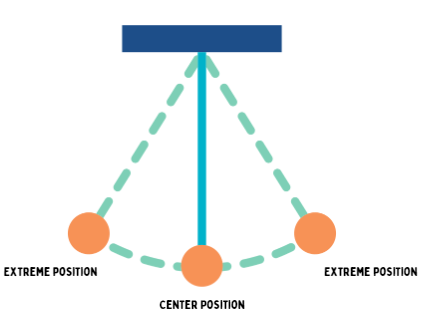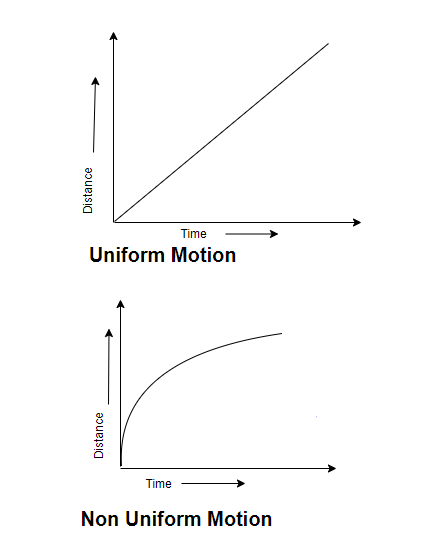# Motion and Time Class 7 Notes

In this page we have Motion and Time Class 7 Notes . Hope you like them and do not forget to like , social share and comment at the end of the page.

## Introduction

Motion can be defined as the change of position of an object with respect to time. In simple words, a body is said to be in motion if it’s position changes with time. Motion is measured as the distance covered by an object in a specific interval of time.

## Scalar and Vector Quantities

A physical quantity which has only magnitude but no direction is called scalar quantity. Examples- Length, Distance, Area, Mass, Time, Energy etc.
A physical quantity which has both magnitude and direction is called a vector quantity. Examples- Displacement, Velocity, Acceleration, Force, Weight etc.

## Different types of motion

Periodic motion -The motion which repeats itself after fixed intervals of time.
Rolling motion- A combination of rotational and translational motion.
Rotatory motion- The motion that occurs when a body rotates on its own axis.
Oscillators and Vibratory motion – The to and fro motion of the body about its fixed position.
Translatory motion – A motion in which all points of a moving body move uniformly in the same direction.

## Slow and fast motion

Fast and slow motion can be defined as the how fast or slow an object is moving. If an object takes a long time go cover a certain distance is known as slow while an object which takes shorter time to cover the same distances is known as fast. This can be represented by the speed of the moving object.
Speed= Distance covered by the time taken or It can be defined as the distance covered in unit time.
The SI unit of speed is metre per second.
The larger values of speed are expressed in kilometre per hour (km/h) whereas the small values of speed are expressed in centimetre per second (cm/s).

## Instrument for measuring speed

SPEEDOMETER
The speed of a moving vehicle is taken in a device called speedometer.
ODOMETER
A device used for measuring the distance covered by a vehicle is known as odometer.
Example
A bus travels 54 km in 90min.What will be the speed of a bus?
Firstly, change the units to m/s
As we know,
Speed = Distance/Time=54×1000/901000 =10m/s.
Note – If we have to compare the speeds of number of objects, then we must express the speeds of all those objects in the same unit.

## Uniform and Non-Uniform motion

When a body covers equal distances in equal intervals of time, then the body is said to be in uniform motion whereas, when a body moves unequal distances in equal intervals of time or vice versa, then it is said to be in non-uniform motion.

## Time

A duration in which things occur is known as time. In ancient time, periodic events such as sunrise, sunset, and phases of the moon were used for measuring time intervals.
So.the time between one sunrise and the next was known as day. And to find month,time from one full moon to the next full moon was considered as month.
There were some of the instruments made by humans in ancient days were as follows
Sun dial – It measures time by the position of the sun or by measuring the length of the shadow during the day time but there is one limiting factor in it that it cannot function during cloudy weather and at night.
Water clock- A device which uses the rate at which water drip from one vessel to another measure time interval is known as water clock.
Sand clock- The device which uses the flow of sand from one glass bulb to another in order to measure time is known as sand clock.
But unfortunately, these instruments were not able to give accurate time. The need for accurate time therefore led to invention of pendulum clocks and digital watches.
Quartz clock – The type of watches or clocks having an electric circuit with one or more cells. The time measured by these clocks is much more accurate .
Unit of time
The basic unit of time is second.
Some units to measure time are as follows
60 seconds= 1 minutes
60 minutes = 1 hours
24 hours = 1 days
365 days = 1 years

## Simple pendulum

A simple pendulum consists of a small metal ball called Bob which is suspended by a long thread from rigid support such that bob is free to swing back and forth. Galileo was the first to experiment with pendulum and his invention made the measurement of time accurate.The development of a simple pendulum made the measurement of time accurate. A simple pendulum is an example of oscillatory motion in which pendulum moves to and fro or back and forth. The pendulum is said to have complete one oscillation when its Bob starting from one mean position to back. The pendulum also completes one oscillation when its bob moves from one extreme position to the other. The time Period of the pendulum depends on its length.
Oscillation
It can be defined as one complete to and fro motion of a pendulum.
Time Period
The time after which the motion repeats itself or it can be defined as the time taken by a pendulum to complete one oscillation. It can be written as-
Time Period (T)= Total time taken/No. of oscillations or vibrations.
THE SI unit of time is second.
A simple pendulum takes 32 s to complete 20 oscillations, what is the time Period of the pendulum?
Time taken to complete 20 oscillations= 32 s
Time taken to complete one oscillation= 32/20 s = 1.6 s
So, Time Period of pendulum is 1.6 seconds

## Graphical Representation of Motion

A distance time graph represents how the distance travelled by a moving object change with time.
Distance-Time Graph
Characteristics of distance – time graph for the motion of an object moving with a constant speed is a straight line inclined on x- axis at some angles. In distance – time graph, distance is always taken along the y- axis while the time is taken along the x- axis.
The distance- time graph easily interpret whether the given object is having uniform or non- uniform motion.
The distance- time graphs tell the distance covered by an object in a given interval of time.
The distance-time graph can be also be made in the form of pie charts and bar graphs.
If the distance- time graphs of two or more objects are plotted in the same graph, then we can compare their speeds.Slope of the graph or gradient
The inclination that the line of graph makes with the horizontal or the x-axis is called slope of the graph or gradient.
Slope of the graph calculate the speed of the distance- time graph.
The steeper the slope of the graph the more is the speed of object.

## Summary

Here is Motion and Time Class 7 Notes
• The distance moved by an object in a unit time is called its speed and it help us to decide which one is moving faster than the other.Unit of speed is metre per second (m/s). 
• Periodic events are used for the measurement of time. Periodic motion of a pendulum has been used to make clocks and watches. 
• The distance-time graph for the motion of an object moving with a constant speed is a straight line

li>Notes
• NCERT Solutions
• Assignments